# 聚类算法学习

Posted by jjx on December 11, 2016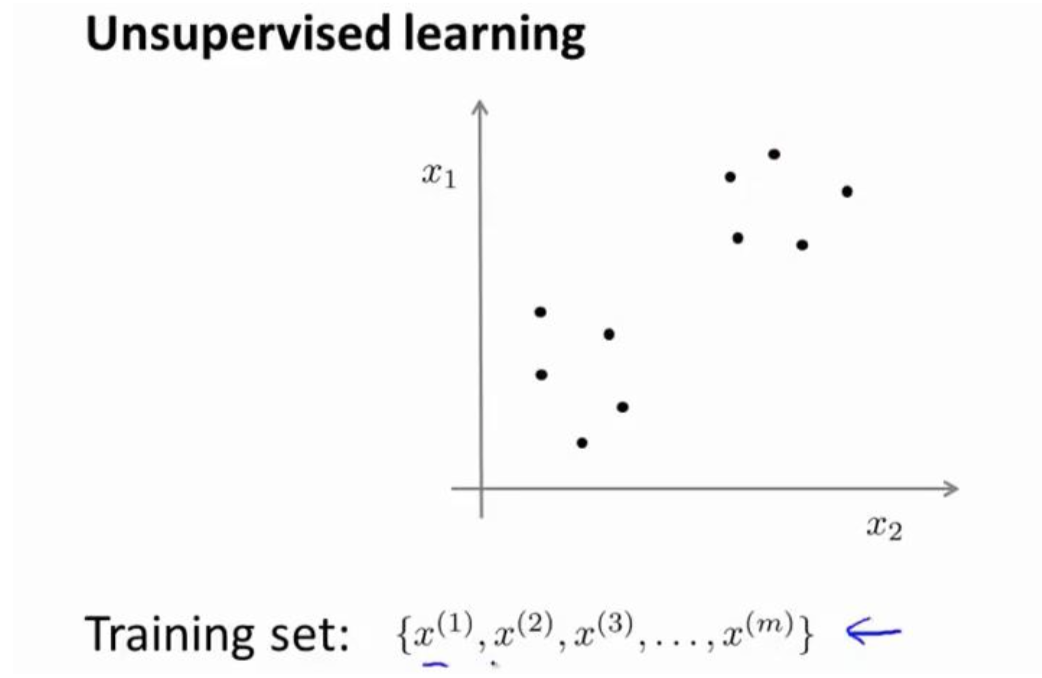K-均值算法
K-均值是最普及的聚类算法,算法接受一个未标记的数据集,然后将数据聚类成不同的 组。
K-均值是一个迭代算法,假设我们想要将数据聚类成 n 个组,其方法为:

• 首先选择 K 个随机的点,称为聚类中心(cluster centroids);
• 对于数据集中的每一个数据,按照距离 K 个中心点的距离,将其与距离最近的中心点关 联起来,与同一个中心点关联的所有点聚成一类。
• 计算每一个组的平均值,将该组所关联的中心点移动到平均值的位置。
• 重复步骤 2-4 直至中心点不再变化。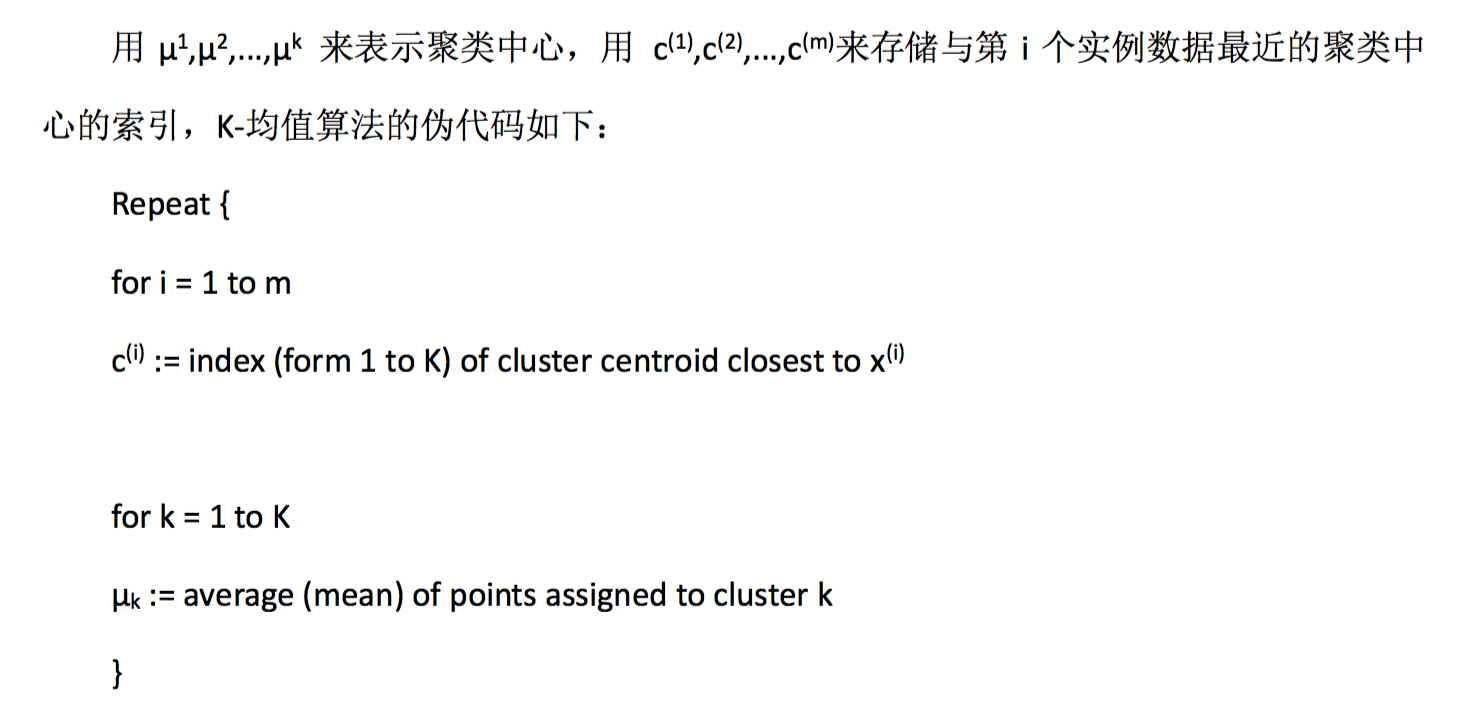K-均值算法也可以很便利地用于将数据分为许多不同组,即使在没有非常明显区分的组 群的情况下也可以。下图所示的数据集包含身高和体重两项特征构成的,利用 K-均值算法将 数据分为三类,用于帮助确定将要生产的 T-恤衫的三种尺寸。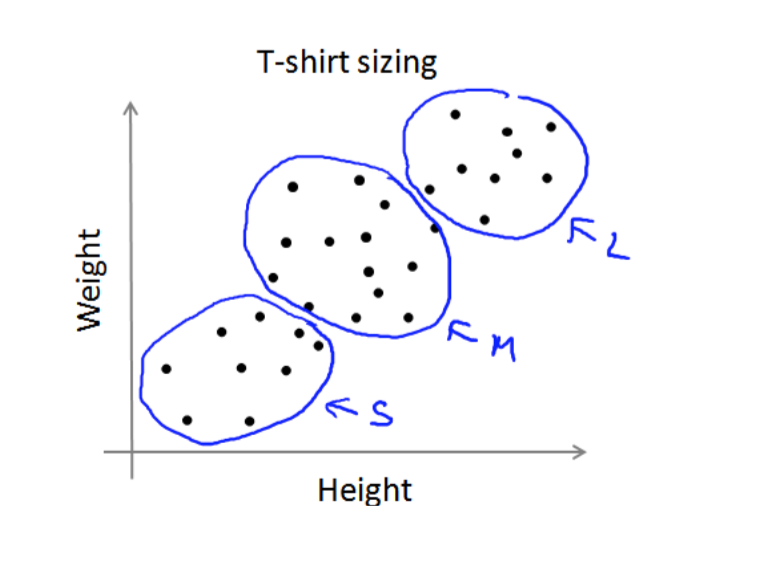K-均值最小化问题,是要最小化所有的数据点与其所关联的聚类中心点之间的距离之和, 因此 K-均值的代价函数(又称畸变函数 Distortion function)为: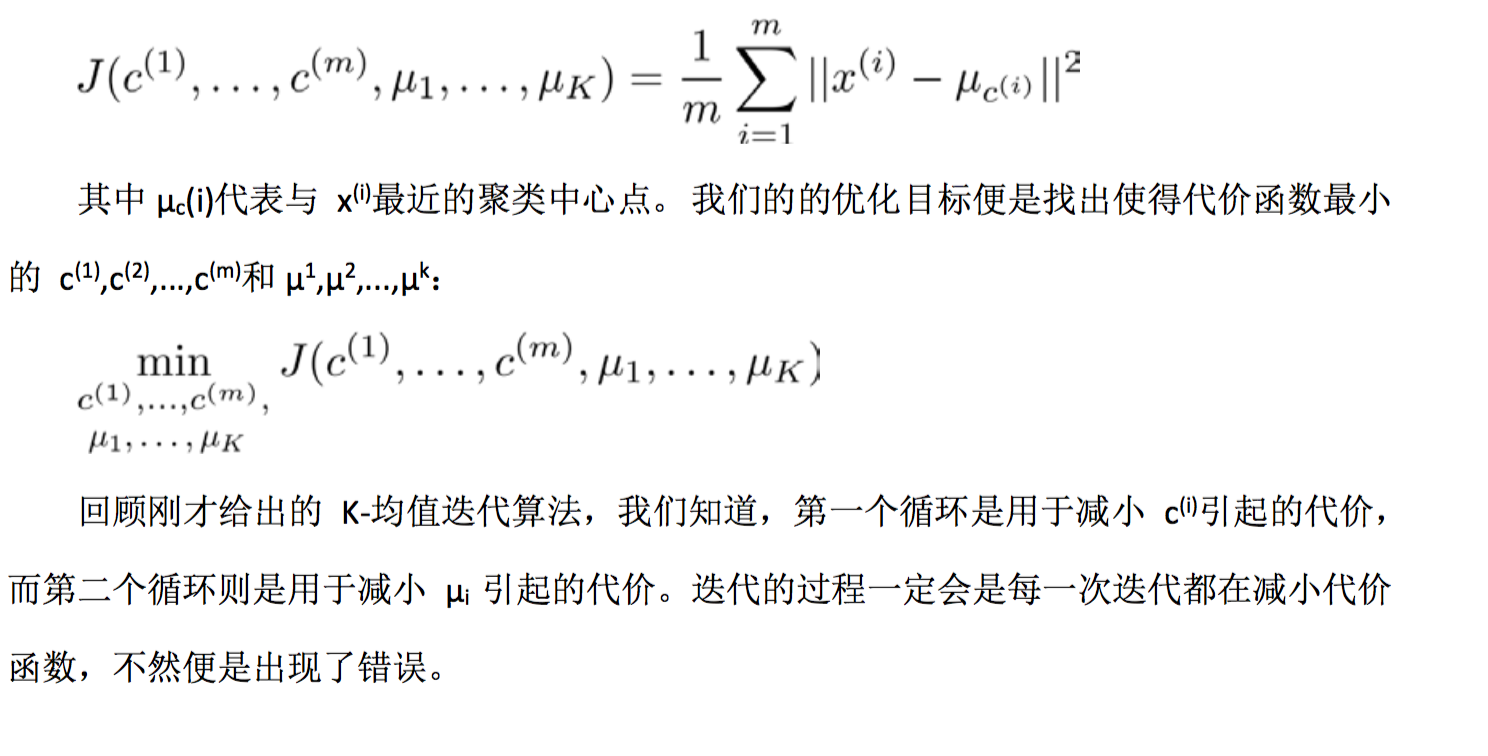1. 我们应该选择 K<m,即聚类中心点的个数要小于所有训练集实例的数量
2. 随机选择 K 个训练实例,然后令 K 个聚类中心分别与这 K 个训练实例相等

K-均值的一个问题在于,它有可能会停留在一个局部最小值处,而这取决于初始化的情 况。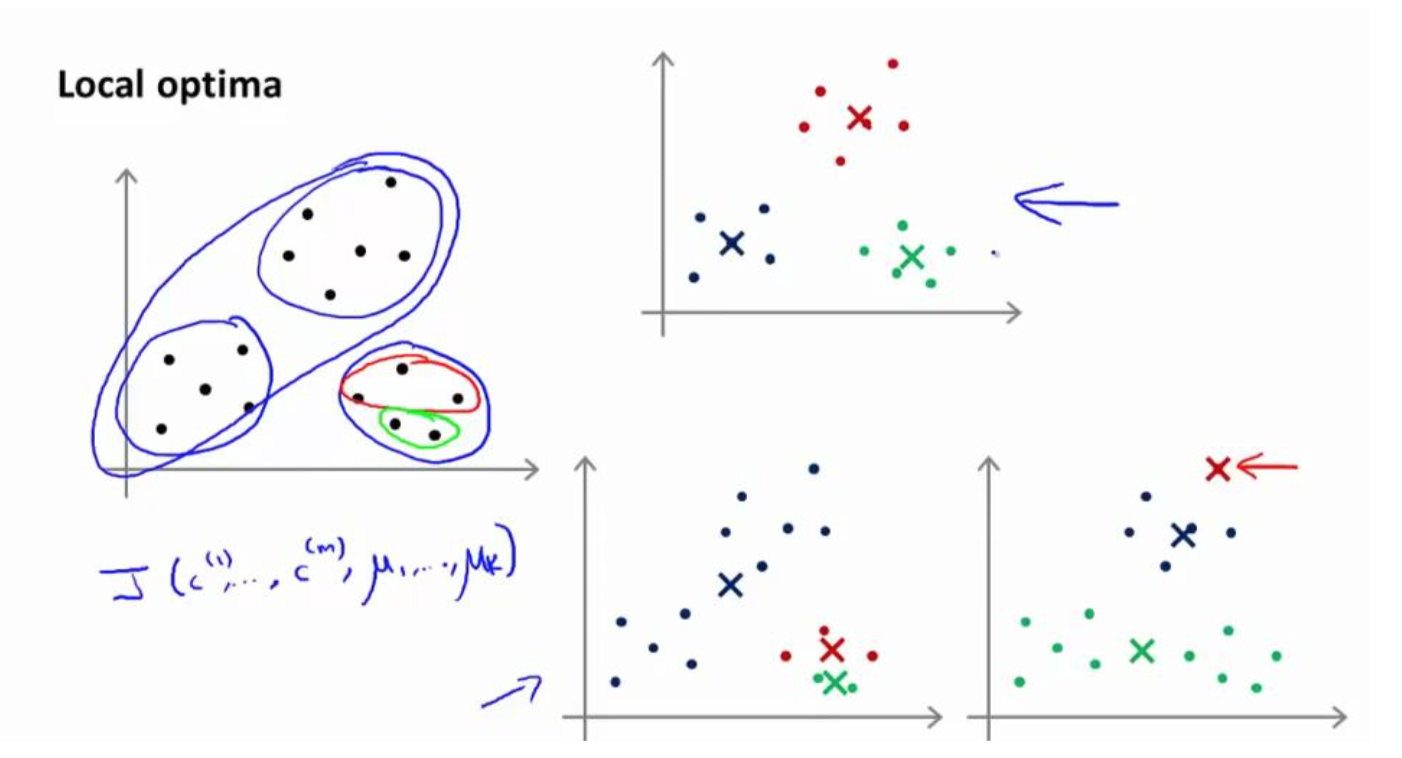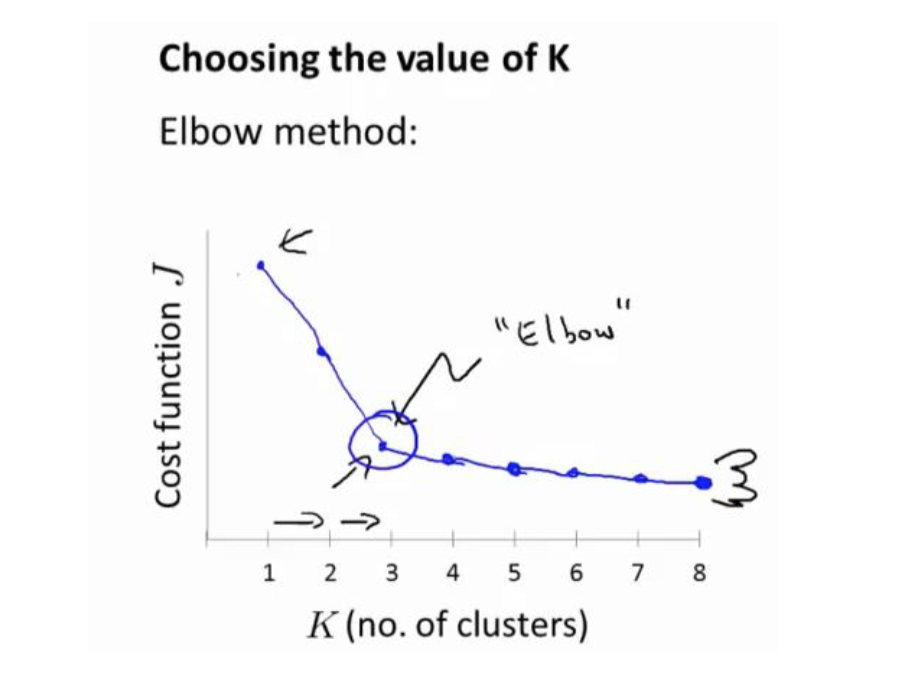function idx = findClosestCentroids(X, centroids)

% Set K
K = size(centroids, 1);

% You need to return the following variables correctly.
idx = zeros(size(X,1), 1);

for i=1:length(idx)
distanse = pdist2(centroids,X(i,:));   % compute the distance(K,1)   pdist2 is a good function
[C,idx(i)]=min(distanse);           % find the minimum
end
end


function centroids = computeCentroids(X, idx, K)
for i=1:K
centroids(i,:) =  mean( X( find(idx==i) , :) );   %
end
end